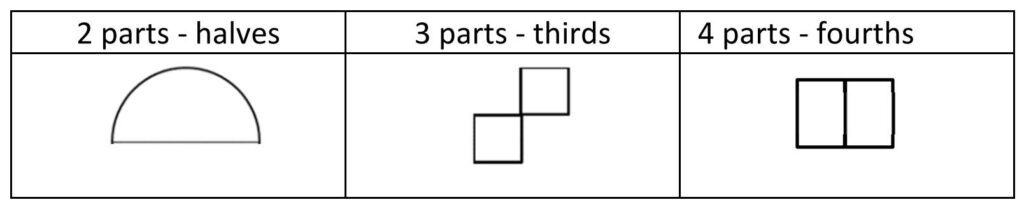# 2nd Grade, Activity 376: Reason with Shapes and their Attributes

Partition circles and rectangles into two, three, or four equal shares, describe the shares using the words halves, thirds, half of, a third of, etc., and describe the whole as two halves, three thirds, four fourths. Recognize that equal shares of identical wholes need not have the same shape(Shapes and Attributes).

### Complete the Drawing

• Group: Alone
• Materials: Complete the Drawing sheet

Write the examples below on the board. Explain that students will partition circles and rectangles into two, three, and four equal shares of halves, thirds, and fourths. Discuss the number of parts and associate the parts with appropriate fractional phrases. Invite students to the board to complete drawings to form halves, thirds, and fourths. Make sure students understand that all the parts of the drawings must be equivalent.Issue the Complete the Drawing sheet to each student. Explain that a partial model is given along with the number of equal parts for the whole model. Instruct students to complete the drawings to show the missing parts(Shapes). Have a discussion to allow students to describe the parts using the words halves, thirds, and fourths.